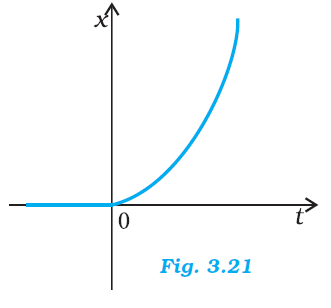## Pages

### Motion in a straight line NCERT Solutions Class 11 Physics - Solved Exercise Question 3.17

Question 3.17

Figure 3.21 shows the x-t plot of one-dimensional motion of a particle. Is it correct to say from the
graph that the particle moves in a straight line for t < 0 and on a parabolic path for t >0 ? If not, suggest a suitable physical context for this graph.Solution:

No
The x-t graph of a particle moving in a straight line for t < 0 and on a parabolic path for t > 0 cannot be shown as the given graph. This is because, the given particle does not follow the trajectory of path followed by the particle as t = 0, x = 0. A physical situation that resembles the above graph is of a freely falling body held for sometime at a height